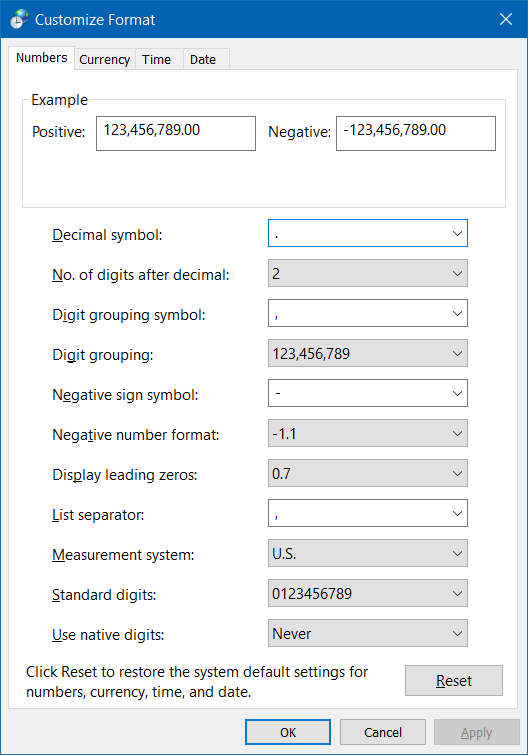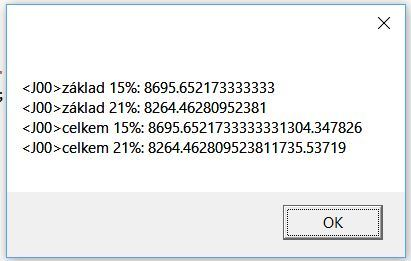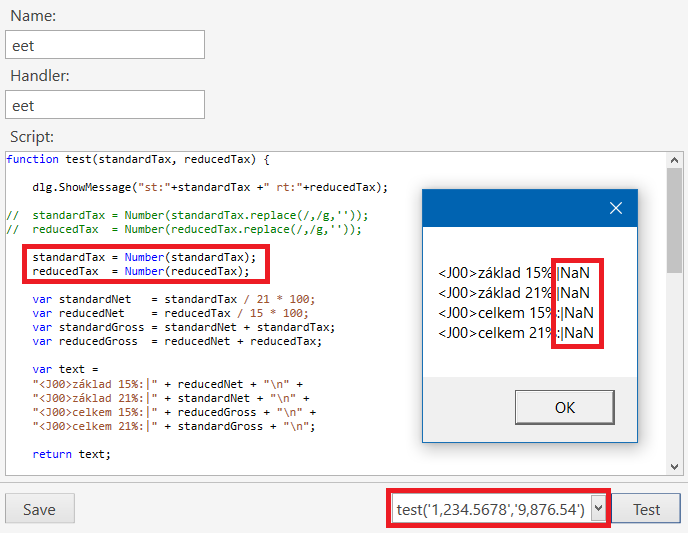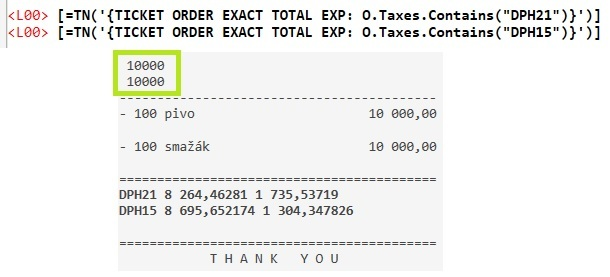# Access tax base, tax rate and total tax in ticket template

I need to call a script from within a ticket template with the following parameters (among others): Tax1 Rate, Tax1 Base, Tax1 Total, Tax2 Rate, Tax2 Base, Tax2 Total. Getting this numbers is easy in the [TAXES] section with {TAX RATE}, {TAXABLE AMOUNT} and {TAX AMOUNT} but I need them all at once.
I figured out how to get the tax amount - {TAX AMOUNT:Tax1} works perfectly anywhere in the template. I’ve been trying hard to get the other numbers with no luck at all. There is a workaround - get the tax amount and use simple math to calculate the taxable amount and hard code the tax rates but I don’t want to use this solution for obvious reasons.
I’m sure there is a nice and clean solution and I’m just missing something. Any help would me much appreciated.
Thanks,
stroodl

1 Like

You would be easily able to use SQL to get the tax rate so if you input tax1 and tax2 amounts, get rate inside script with sql you can then easily calculate the taxable amount.
So long as you put the tax amount into the script in the same order you can do the rest inside the scipt.

Thank you for the suggestion but thats exactly what I wanted to avoid - it’s like crossing a river to get water while there has to be a more elegant solution in such a powerful and complex piece of sw (Samba has acces to all the numbers needed as it can be easily displayed under the [TAXES] section).
Anyway, I tried calculating the tax bases in a script and I might have found another bug: Calling a simple script with two parameters {CALL:eet.test({TAX AMOUNT:21%DPH},{TAX AMOUNT:15%DPH})} returns wrong results as the tax amount is a string with comma separated “decimals” which messes up the parameters. What I need to do is to convert it to a number first but the ToNumber function does not seem to work with {TAX AMOUNT:X}
[=TN(’{TAX AMOUNT:21%DPH}’)] gives me e.g. “12 345,00” while [=TN(’{TICKET TOTAL}’)] would return 12345.00

Pretty sure you need quotes arround your {TAX AMOUNT:xx} tags in the CALL.
Maybe if you can elaborate on what your doing in the script an alternative solution might be available, all we know at the minute is your wanting to input all the tax details into a script. THere might be a better route to take.

The script uses an external application that sends an xml data message to local financial authority server that records each recepit and returns it’s unique code which has to be printed on the receipt. The message must contain bases and taxes for all three VAT’s plus some other info. Everything works well (thanks to a friend of mine who did an incredible job) - calling the script with hard-coded data from within a ticket template prints out the receipts’ unique code or stores the data in a database if the connection fails.
The very last step on this long and exhausting journey is to call the script with real data which was believed to be a super easy task.
I’m afraid there’s no workaround - what I need to do is to call a script from within a ticket template with tax1 base, tax1 amount, tax2 base, tax2 amount as parameters.
EDIT: I tried adding quotes around {TAX AMOUNT:X} - it gives me an Not a Number exception.
Here is my test script:
function test(standardTax, reducedTax) {

var standardNet = standardTax / 21 * 100;
var reducedNet = reducedTax / 15 * 100;
var standardGross = standardNet + standardTax;
var reducedGross = reducedNet + reducedTax;

var text =
"základ 15%: " + reducedNet + “\n” +
"základ 21%: " + standardNet + “\n” +
"celkem 15%: " + reducedGross + “\n” +
"celkem 21%: " + standardGross + “\n”;

return text;
}

and the call:
{CALL:eet.test({TAX AMOUNT:21%DPH},{TAX AMOUNT:15%DPH})}

``````<F>=
<EB>
<W00:27, 2, 9, 3, 7>Total:|L|[=F((TN('{TICKET TOTAL}')-TN('{CALCULATION TOTAL: }'))/TN('{EXCHANGE RATE:HNL}'))]|\$|[=F(TN('{TICKET TOTAL}')-TN('{CALCULATION TOTAL: }'))]
<DB>
<F>=
<EB>
<W00:27, 2, 9, 3, 7>Subtotal:|L|[=F((TN('{TICKET TOTAL}')-TN('{TAX TOTAL}')-TN('{CALCULATION TOTAL:Round HNL +}'))/TN('{EXCHANGE RATE:HNL}'))]|\$|[=(F(TN('{TICKET TOTAL}')-TN('{TAX TOTAL}')-TN('{CALCULATION TOTAL:Round HNL +}')))]
<DB>
{TAXES}
<EB>
<W00:27, 2, 9, 3, 7>Tax Total:|L|[=F(TN('{TAX TOTAL}')/TN('{EXCHANGE RATE:HNL}'))]|\$|[=F('{TAX TOTAL}')]
<DB>
{SERVICES}
<EB>
<W10:27, 2, 9, 3, 7>GRAND TOTAL:|L|[=F(Math.round(TN('{TICKET TOTAL}')/TN('{EXCHANGE RATE:HNL}')))]|\$|[=F(TN('{TICKET TOTAL}'))]
<DB>

[TAXES]
<W00:27, 2, 9, 3, 7>Tax {TAX NAME} ({TAX RATE}%):|L|[=F(TN('{TAX AMOUNT}')/TN('{EXCHANGE RATE:HNL}'))]|\$|[=F('{TAX AMOUNT}')]

[SERVICES]
<W00:27, 2, 9, 3, 7>{CALCULATION NAME} ([=F(TN('{CALCULATION AMOUNT}'))]):|L|[=F(TN('{CALCULATION TOTAL}')/TN('{EXCHANGE RATE:HNL}'))]|\$|[=F('{CALCULATION TOTAL}')]
``````

Produces:

``````================================================
Total:                      L   621.00  \$  27.00
================================================
Subtotal:                   L   535.93  \$  23.30
Tax T1 (15%):               L    57.00  \$   2.48
Tax T2 (18%):               L    28.07  \$   1.22
Tax Total:                  L    85.07  \$   3.70
GRAND TOTAL:                L   621.00  \$  27.00
``````

You can see the SubTotal is produced with something like this, which should be the same as `{PLAIN TOTAL}`, but may not be for various reasons:

``````[=TN('{TICKET TOTAL}')-TN('{TAX TOTAL}')-TN('{CALCULATION TOTAL:Round HNL +}')]
``````

What are your Regional Settings in Windows for thousands separator and decimal separator?Are you over-riding Regional Settings in SambaPOS?

JScript also has API `Helper.ToNumber()` function which should do the same thing as `TN()`.

This can be done with JScript for sure, and since you are sending results using JScript, it is a natural place to do this.

Give some hard number examples of exactly what is in each of the following as raw text, including thousands and decimal separators, whether they be comma, dot, spaces, etc:

``````{TAX AMOUNT:21%DPH}
{TAX AMOUNT:15%DPH}
{TAX TOTAL}
{TICKET TOTAL}
``````

These may also be helpful to you since they are Ticket-Level Tags:

``````PLAIN TOTAL Ticket Sub Total
PLAIN TOTAL:X Ticket Sub Total
``````

With actual string/text output of those Tags, we can use the Test facility in the JScript to see exactly what is going on, and manipulate the function parameters as needed …

1 Like

Thank you for your help. I changed the Windows regional settings to use ‘.’ as a decimal point and set no Digit grouping but this does not seem to affect Samba (I do not override Windows Regional Settings in Samba).
I’ve created a sample ticket and added the tags to template. Here is what i get:

{TAX AMOUNT:21%DPH} prints as 1 304,347826
{TAX AMOUNT:15%DPH} prints as 1 735,53719
{TAX TOTAL} prints as 3 039,885016
{TICKET TOTAL} prints as 20 000,00

I also edited the test function according to your suggestion and fired test(‘1 735,53719’,‘1 304,347826’):

function test(standardTax, reducedTax) {

standardTax = standardTax.replace(/ /g,’’).replace(/,/g,’.’);
reducedTax = reducedTax.replace(/ /g,’’).replace(/,/g,’.’);
//1 return standardTax;
//3 return Helper.ToNumber(standardTax);

var standardNet = standardTax / 21 * 100;
var reducedNet = reducedTax / 15 * 100;
var standardGross = standardNet + standardTax;
var reducedGross = reducedNet + reducedTax;

var text =
"základ 15%: " + reducedNet + “\n” +
"základ 21%: " + standardNet + “\n” +
"celkem 15%: " + reducedGross + “\n” +
"celkem 21%: " + standardGross + “\n”;
//2 return text;
}

uncommenting //1 returns 1735.53719
So far so good. However, uncommenting //2 returns this:uncommenting //3 returns 0

Is the grouping a space and decimal is comma?

That makes no sense, given this:

Those settings should affect SambaPOS. Restart the Computer or definitely restart SambaPOS and post your results again please.

So, the ToNumber function does not like your regional format… 'nuff said, we don’t need to use it. But we do need to be able to make a solid assumption about the format of the incoming numbers…

``````function test(standardTax, reducedTax) {

var o = standardTax;
var r = standardTax.replace(/ /g,'').replace(/,/g,'.');
var h = Helper.ToNumber(r);
var n = Number(r);

var msg1 = "o:"+o +"\r\nr:"+r +"\r\nh:"+h +"\r\nn:"+n;

var o = reducedTax;
var r = reducedTax.replace(/ /g,'').replace(/,/g,'.');
var h = Helper.ToNumber(r);
var n = Number(r);

var msg2 = "o:"+o +"\r\nr:"+r +"\r\nh:"+h +"\r\nn:"+n;

return msg1 + "\r\n\r\n" + msg2;

var standardNet = standardTax / 21 * 100;
var reducedNet = reducedTax / 15 * 100;
var standardGross = standardNet + standardTax;
var reducedGross = reducedNet + reducedTax;

var text =
"základ 15%: " + reducedNet + "\n" +
"základ 21%: " + standardNet + "\n" +
"celkem 15%: " + reducedGross + "\n" +
"celkem 21%: " + standardGross + "\n";

return text;

}
``````

Return:

``````o:1 735,53719
r:1735.53719
h:1735.53719
n:1735.53719

o:1 304,347826
r:1304.347826
h:1304.347826
n:1304.347826
``````

The `replace()` function gives us what we want, though we should also ensure it is a number rather than a string, so using `Helper.ToNumber()` or simply `Number()` after `replace()` will make that happen… then we can do the rest of the math… is this correct? Looks good to me…

Return:

``````základ 15%: 8695.652173333333
celkem 15%: 9999.999999333333
celkem 21%: 9999.99999952381
``````

Script:

``````function test(standardTax, reducedTax) {

standardTax = Number(standardTax.replace(/ /g,'').replace(/,/g,'.'));
reducedTax  = Number(reducedTax.replace(/ /g,'').replace(/,/g,'.'));

var standardNet   = standardTax / 21 * 100;
var reducedNet    = reducedTax / 15 * 100;
var standardGross = standardNet + standardTax;
var reducedGross  = reducedNet + reducedTax;

var text =
"základ 15%: " + reducedNet + "\n" +
"základ 21%: " + standardNet + "\n" +
"celkem 15%: " + reducedGross + "\n" +
"celkem 21%: " + standardGross + "\n";

return text;

}
``````

You were right - restarting pc did the trick, Samba now uses the newly set formatting. I don’t feel like changing this on my live setup as it might bring more problems than it solves.
but this seems to do the job:

standardTax = standardTax.replace(/ /g,’’).replace(/,/g,’.’);
standardTax = Number(standardTax);

test(‘1 735,53719’,‘1 304,347826’) returns the correct results.
However, calling {CALL:eet.test(’{TAX AMOUNT:21%DPH}’,’{TAX AMOUNT:15%DPH}’)} from within a ticket template returns Not a Number exception.

You have hit the quirk about using Scripts. Using the Test facility, your changes are reflected immediately in your results, but not when feeding values from a Template (at least in Template Preview mode).

If you make a change in the Script, and you want to test using values from your Template (Preview), you will need to Save the Script, then Logout and Login to reload the cache, because it is still using the “old” script.

Frustrating, I know. @emre?

BTW, if you want all those lines printed on the Ticket, you need to change your return. To get this:

Notice there is no leading Tag (ie. `<L00>` or `<J00>`, etc). We supply those in the Script:``````function test(standardTax, reducedTax) {

standardTax = Number(standardTax.replace(/ /g,'').replace(/,/g,'.'));
reducedTax  = Number(reducedTax.replace(/ /g,'').replace(/,/g,'.'));

var standardNet   = standardTax / 21 * 100;
var reducedNet    = reducedTax / 15 * 100;
var standardGross = standardNet + standardTax;
var reducedGross  = reducedNet + reducedTax;

var text =
"<J00>základ 15%:|" + reducedNet + "\n" +
"<J00>základ 21%:|" + standardNet + "\n" +
"<J00>celkem 15%:|" + reducedGross + "\n" +
"<J00>celkem 21%:|" + standardGross + "\n";

return text;

}
``````

The fundamental question is, whether the `{TAXABLE AMOUNT:XXX}` can be used outside of the `[TAXES]` section. It seems that it cannot. But can it be implemented in a future version. Apart from the need to calculate the taxable amount through the tax rate taken from the DB (or hard-coded), the multiplication result does not match the original value due to the rounding error.

Only @emre can answer that question. But if we can get `{TAX AMOUNT:X}` in the Ticket section (`[LAYOUT]`), I don’t see why we couldn’t have access to `{TAXABLE AMOUNT:X}` and `{TOTAL TAXABLE AMOUNT}` as well. And for that matter, I don’t understand why any/all of the Tags in the `[TAXES]` section could not be available in the Ticket section.

Not sure what you mean here. You can round your numbers before, during, or after calculation(s), and it appears you have used sufficient decimal places to make it accurate. Can you give an example?

I restarted the pc, settled a new ticket and I’m still getting the Not a Number error:

I think we can make this work but what I need is as simple, robust and reliable solution as possible. Making a single mistake in the xml message could bring me into serious troubles with financial authorities.
Taking a number (tax amount) from database, format it as a string, then use a script to replace some characters, format it back to a number to use it to calculate a tax base, somehow deal with a possible rounding error and all of this just to get a number that Samba can return with the {TAXABLE AMOUNT:XXX} tag- this is just too complicated solution to my taste.

Ok, let’s check one more thing… put this as your first line in the `eet.test()` script, and execute the Print Preview:

``````dlg.ShowMessage("st:"+standardTax +" rt:"+reducedTax);
``````

Remember to Logout/Login after saving the script.

When you execute Print Preview, you should see a Message Box appear:

I have this sneaking suspicion your Tax Template Names might be causing parsing troubles because of the `%` symbol in them.

Message Box works fine. I also renamed the tax templates to DPH15 and DPH21 but still getting the NaN.
To summarize:
{TAX AMOUNT:DPH21} prints 1 735,53719 on the ticket, DPH15 works as well
running test(‘1 735,53719’,‘1 304,347826’) returns the correct values
but {CALL:eet.test(’{TAX AMOUNT:DPH21}’,’{TAX AMOUNT:DPH15}’)} returns NaNWill get back tomorrow. Thank you for your time and effort.

Ok, well now I am wondering about your Regional Settings as they are being applied to the JScript Engine.

If the JScript Engine is aware that your grouping symbol is space and your decimal separator is comma, then maybe we should NOT be manipulating the function parameters.

At the very least, maybe we should not change the decimal separator from comma to dot, but IIRC, the `Number()` function might not like grouping symbols, so we might still replace those with nothing.

It seems to me that I needed to remove grouping symbols at the very least. This test shows that:

##`Number()` without grouping symbol replacement (fails):##`Number()` with grouping symbol replacement (succeeds):

##`Helper.ToNumber()` without grouping symbol replacement (succeeds):

##Template (using either of the last 2 succeeding methods):

Doesn’t taxable amount is equal to something like…

``````{TICKET ORDER EXACT TOTAL EXP:O.Taxes.Contains("%18")}
``````

or maybe if you need a total of some taxes…

``````{TICKET ORDER EXACT TOTAL EXP:O.Taxes.Contains("%18") or O.Taxes.Contains("%8")}
``````

PS: %18 or %8 can be used as tax template names. It works for me as my tax template names contains tax rates. If you named the template as VAT you can use it like O.Taxes.Contains(“VAT”)

1 Like

First and second methods don’t work for me (since I have a space as a grouping symbol and comma as decimal). You’re right with the third method - JScript is aware of my regional settings:

However, calling {CALL:eet.test(’{TAX AMOUNT:DPH21}’,’{TAX AMOUNT:DPH15}’)} returns NaN.

I also tried [=TN(’{TICKET ORDER EXACT TOTAL EXP: O.Taxes.Contains(“DPH21”)}’)]
This works flawlessly within the template:But putting this expression as a parameter to Call:eet.test() does not call the function at all - the Message Box does not open.
I deleted my database and set everything up from scratch to avoid any errors with the taxes setup (I’m following this tutorial btw: https://sambapos.org/wiki/doku.php/en/how_to_configure_multiple_taxes)

emre’s answer brought me to another idea: since I don’t need to keep the taxes on a separate account I actually dont need the whole tax setup in Samba. Let me simplify my requiremets, we might find a totally different approach:

What I need is:

1. to create 3 groups (VAT1, VAT2, noVAT for takeaway) and put every single product in either VAT1 or VAT2
2. to be able to move a product to the noVAT group when making an order - takeaway order is not subject of this sales record madness
3. to be able to get VAT1 total sum and VAT2 total sum in ticket template and use the data as parameters to call a script
4. to be able to “cancel” the transaction by sending the same data with negative sign.

With tax templates I was able to achieve 1) and 2) (although only oneway but I’m pretty sure I would figure it out)
With item tags I was able to achieve 1) and 3) and 2) might be doable as well, but this solution requires adding TAG for each product (vs. much more convenient tax templates mapping to product groups)

``````{CALL:eet.test('{TICKET ORDER EXACT TOTAL EXP:O.Taxes.Contains("T1")}','{TICKET ORDER EXACT TOTAL EXP:O.Taxes.Contains("T2")}')}
``````

``````základ 15%:                                60000